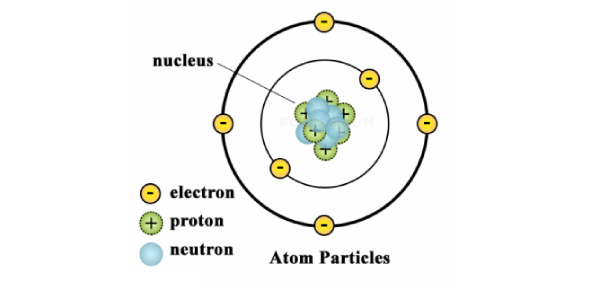# Atoms And Elements Test Quiz!

21 Questions | Attempts: 224Settings.

• 1.
Protons are located in the nucleus of the atom. A proton has:
• A.

No charge

• B.

A negative charge

• C.

A positive and negative charge

• D.

A positive charge

• 2.
Neutrons are in the nucleus of the atom. A neutron has:
• A.

A positive charge

• B.

No charge

• C.

A negative charge

• D.

Twice as much positive charge as a proton

• 3.
An electron is in a region outside the nucleus. An electron
• A.

Is larger than a proton and has no charge

• B.

Has less mass than a proton and has a negative charge

• C.

Is smaller than a proton and has no charge

• D.

Has a positive charge

• 4.
A hydrogen atom is made up of one proton and one electron. The proton and electron stay near each other because:
• A.

Positive and negative charges repel

• B.

Positive and positive charges repel

• C.

Positive and negative charges attract

• D.

Two negatives make a positive

• 5.
The atomic number of an atom is:
• A.

The mass of the atom

• B.

The number of protons added to the number of neutrons

• C.

The number of protons

• D.

Negatively charged

• 6.
The atoms of the same element can have different isotopes. An isotope of an atom:
• A.

Is an atom with a different number of protons

• B.

Is an atom with a different number of neutrons

• C.

Is an atom with a different number of neutrons

• D.

Has a different atomic number

• 7.
The atomic mass of an element is:
• A.

The average mass of all the isotopes of the element

• B.

A measure of the density of that element

• C.

The mass of the most common isotope of that element

• D.

The number of protons and electrons in the atoms of the element

• 8.
An element and an atom are different but related because:
• A.

A particular element is made up of many different types of atoms

• B.

A molecule is the same as an atom

• C.

An element is made up of all the same type of atom

• D.

An element is smaller than an atom

• 9.
The periodic table shows that a carbon atom has six protons. This means that a carbon atom also has:
• A.

Six electrons

• B.

Six neutrons

• C.

More protons than electrons

• D.

An atomic mass that equals six

• 10.
The atomic number of nitrogen is 7. The atomic mass is 14.01. This means that:
• A.

All nitrogen atoms have exactly 7 neutrons

• B.

A small percentage of nitrogen atoms have fewer than 7 neutrons

• C.

A small percentage of nitrogen atoms have more than 7 neutrons

• D.

Some nitrogen atoms have fewer than 7 electrons

• 11.
Electrons are in regions around the nucleus called energy levels. The first energy level:
• A.

Is furthest from the nucleus

• B.

Is closest to the nucleus

• C.

Holds the most electrons

• D.

Needs more than two electrons to fill it up

• 12.
Neon has 10 protons and 10 electrons. The electrons fill the energy levels in Neon like this:
• A.

2 in the first, 2 in the second, and 6 in the third

• B.

4 in the first, 4 in the second, and 2 in the third

• C.

2 in the first, 4 in the second, and 4 in the third

• D.

2 in the first, 4 in the second, and 4 in the third

• 13.
The atoms in a column of the periodic table all have:
• A.

The same abbreviation

• B.

The same abbreviation

• C.

The same number of electrons

• D.

The same number of electrons

• 14.
In the process of covalent bonding, atoms share electrons. This means that:
• A.

Electrons from each atom are attracted to the nucleus of both atoms

• B.

Protons and neutrons attract

• C.

Atoms lose electrons and become ions

• D.

Atoms gain electrons and become ions

• 15.
In the process of ionic bonding:
• A.

Both atoms gain electrons

• B.

One atom gains one or more electrons and the other loses the same numbe

• C.

Atoms switch protons

• D.

Both atoms lose electrons

• 16.
Different atoms of the same element can have a different number of ________
• 17.
The atomic number of an atom is equal to the number of [Blank] in the atom's nucleus.
• 18.
When an atom loses an electron, it forms a [Blank] ion.
• 19.
When an atom gains an electron, it forms a [Blank] ion.
• 20.
In a covalent bond, electrons are [Blank] between two atoms.
• 21.
When an atom loses an electron, it forms a [Blank] ion.

## Related TopicsBack to top
×

Wait!
Here's an interesting quiz for you.